# The concept of functions

In this Mentimeter template, the concept of a function is elaborated on. The template can be used both as an introduction, in the middle or as a form of reinforcement at the end of a subject block surrounding linear functions.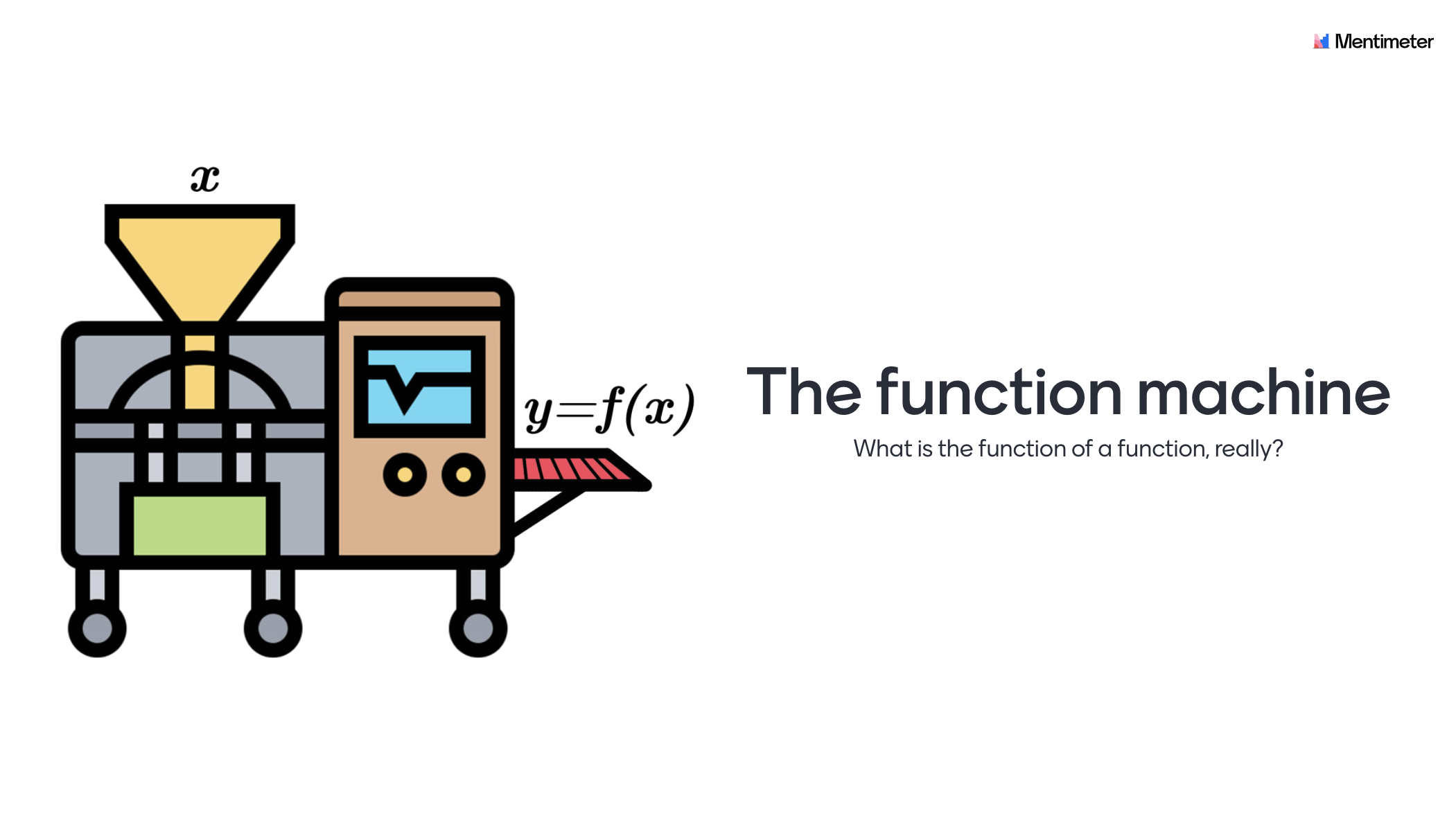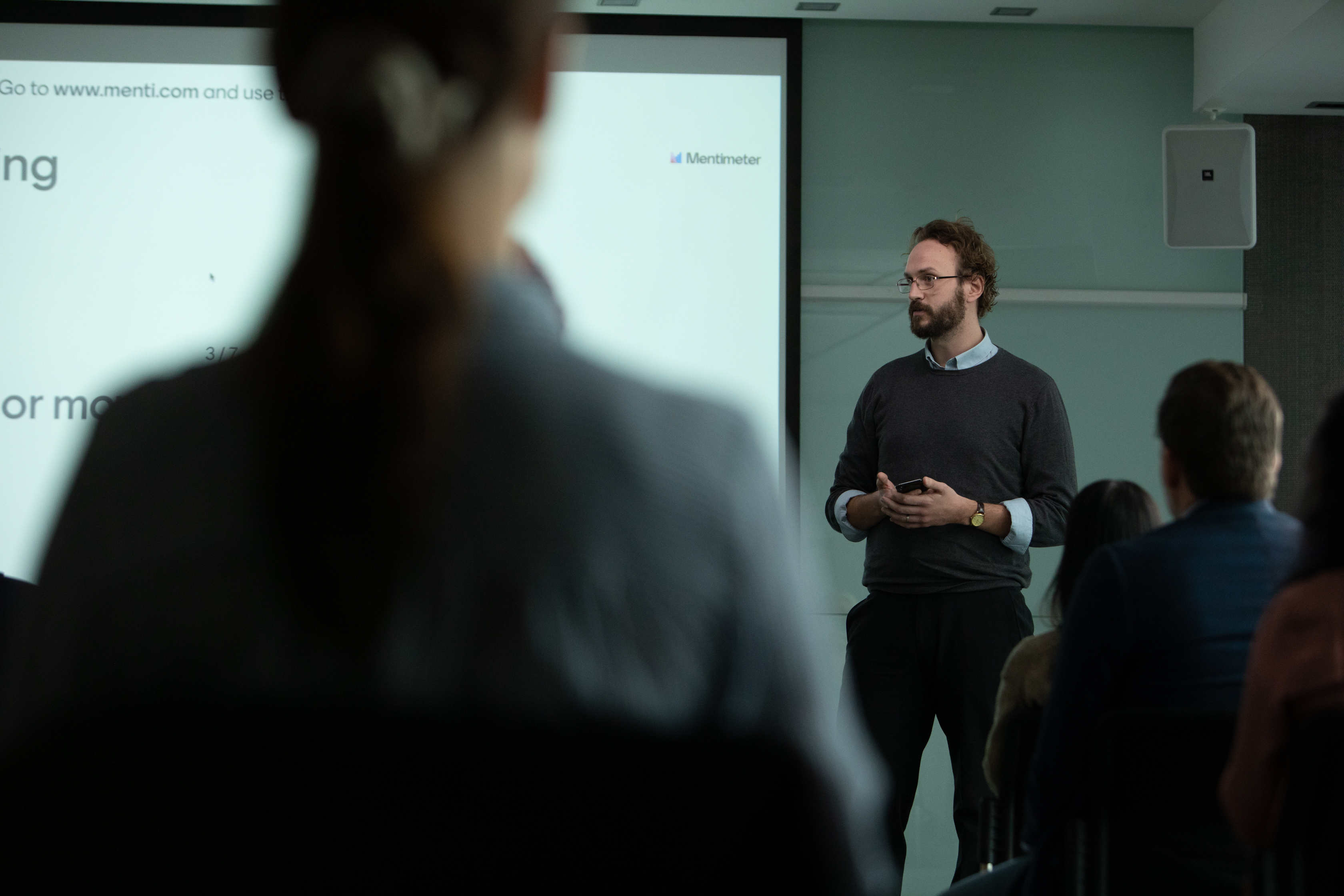## How to use the template

The students need a pencil and paper for some of the questions in the presentation.

Here follows some ideas on how the slides are intended to be used, but feel free to use the template however you like. Included in each section of this page is the purpose and other information that may be of use for you as a teacher. A general idea regarding all slides is that the students should work under a Think-Pair-Share (TPS) model. When implementing TPS the students should first answer the question individually, then discuss in pairs (or groups of three) followed by a classroom discussion. As a concluding remark. The content in the slides are designed primarily for developing the students understanding of mathematical concepts rather than the procedural "know how". Therefore discussions and questions from the students is a central part of using the presentation where your expertise as a teacher is central to clarify misconceptions and lead the classroom discussions.

### Important keyboard shortcuts

To get the most out of presenting with Mentimeter, there are a few keyboard shortcuts you need to know.

Toggle between hiding and showing results: H. It is important to not show results before everyone has had a chance to give their response since this can influence other students' thinking.

Countdown timer: 1 (60 seconds), 8 (30 seconds) 9 (10 seconds), 0 (stop countdown).  It is good to be flexible and to start a timer (either, 60, 30 or 10 seconds) when you feel that the majority of the group is ready to move on.

Show correct answers: ENTER. Wait until all have answered a multiple choice question before you show the correct answers.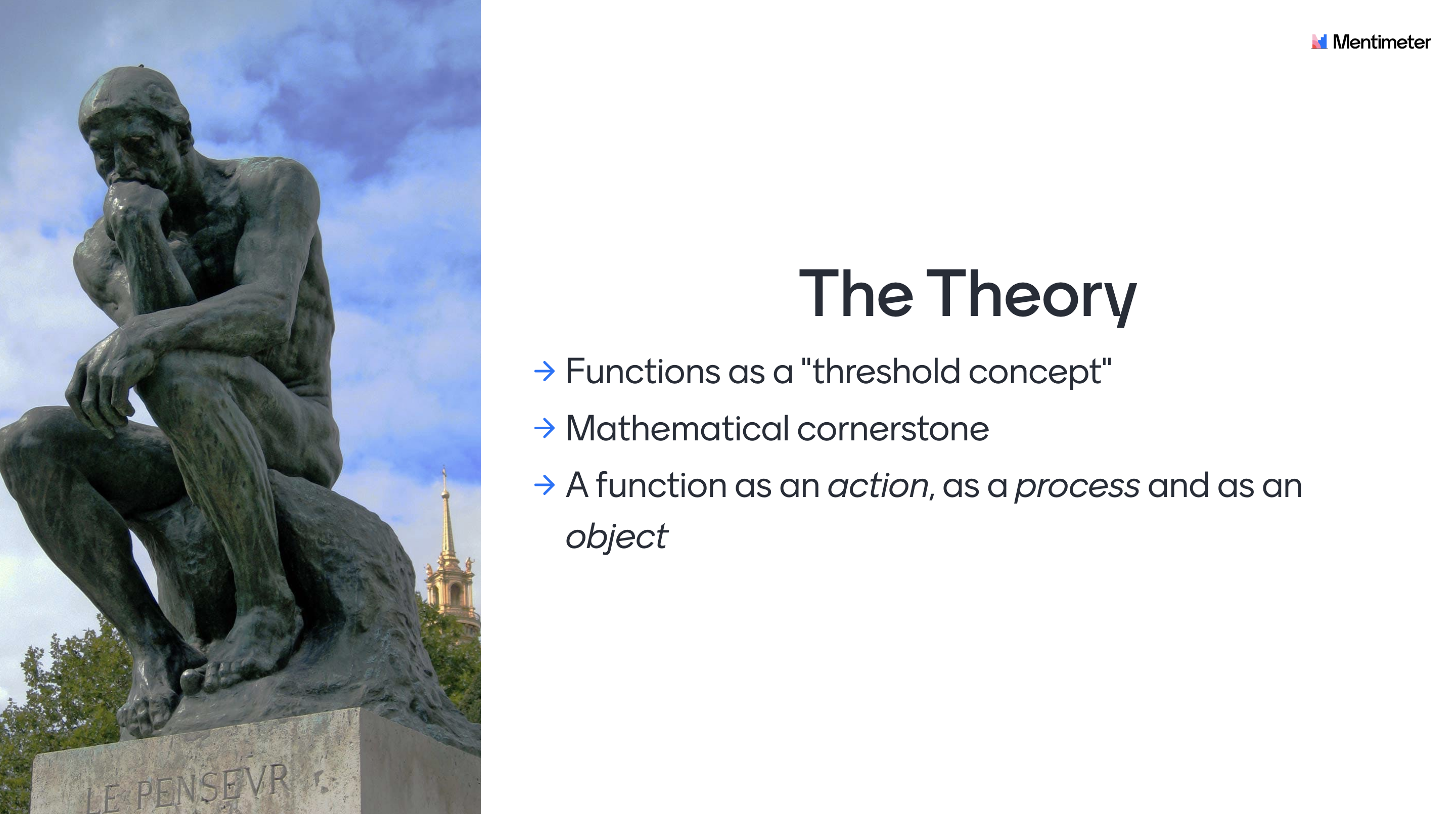## Theoretical background

A function is defined as a threshold concept. This means that it is transformative - once learnt it opens up new ways of conceiving a concept and it will be impossible to forget. Not grasping the concept of a function will hinder the learner to get a rich mathematical understanding later down the line.

To properly grasp the concept of a function, students should be able to conceive of a function as an action, as a process and as an object, all in its own right. The transition that the learner needs to go through when transitioning from conceiving a function as a process, to viewing it structurally as an object, can be very challenging. But once the learner has made this shift in perspective, it is possible to see the construct in a new light and the penny suddenly drops.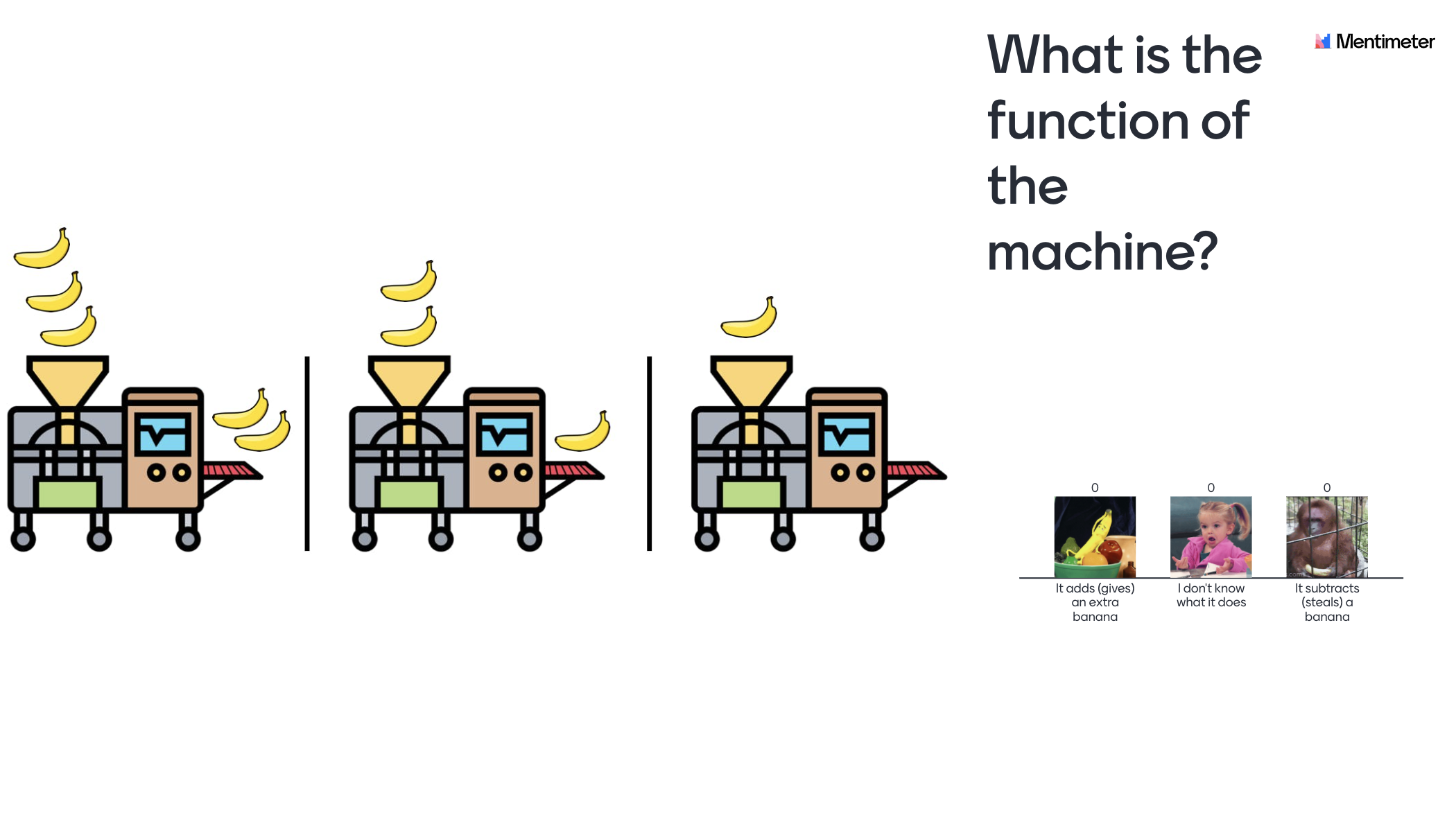Slide 1 - Introduction: Introduce the theme of the lecture. For example, you could mention that the concept of a function is very central to mathematics.

Slide 2 - General information: Inform the students regarding the TPS methodology and how they can access the interactive lesson.

Slide 3 - Criteria for success: Introduce a "criteria for success" for the lesson with a problem that will be revisited and solved at the end of the lesson. The idea here is to make the learning visible, so the students can see for themselves that they’ve actually learned something.

Slide 4 - According to the students, a function is...: Let the students individually write down how they see the concept of a function. This can help clarify potential misconceptions.

Slide 5 - Banana machine 1: A warm up to understand the idea of the "banana-machine".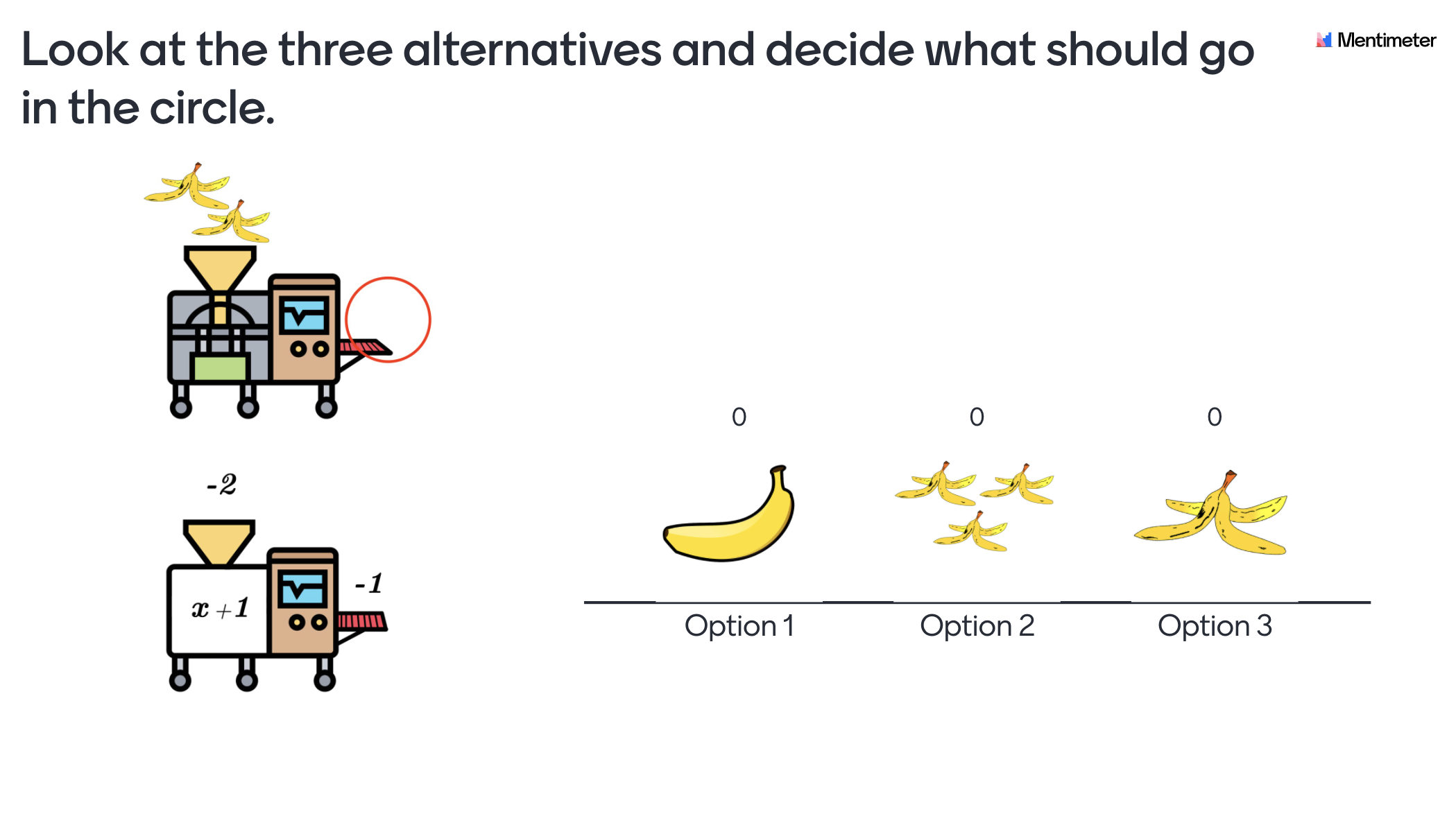Slide 6 - Banana machine 2: Increase the level of abstraction by introducing a half banana.

Slide 7 - Banana machine 3: Get the students to realize that a "machine"/function can contain all the calculation methods (multiplication, division, addition, and subtraction).

Slide 8 - Banana machine 4: Get the students to visualize the "machine"/function in a more abstract manner by introducing negative numbers.

Slide 9-11 - Banana and number machine: These are key slides. The idea here is to go from a more concrete idea using bananas and a machine, to the more abstract mathematical language. Try giving probing questions to the students, even if every group has answered correctly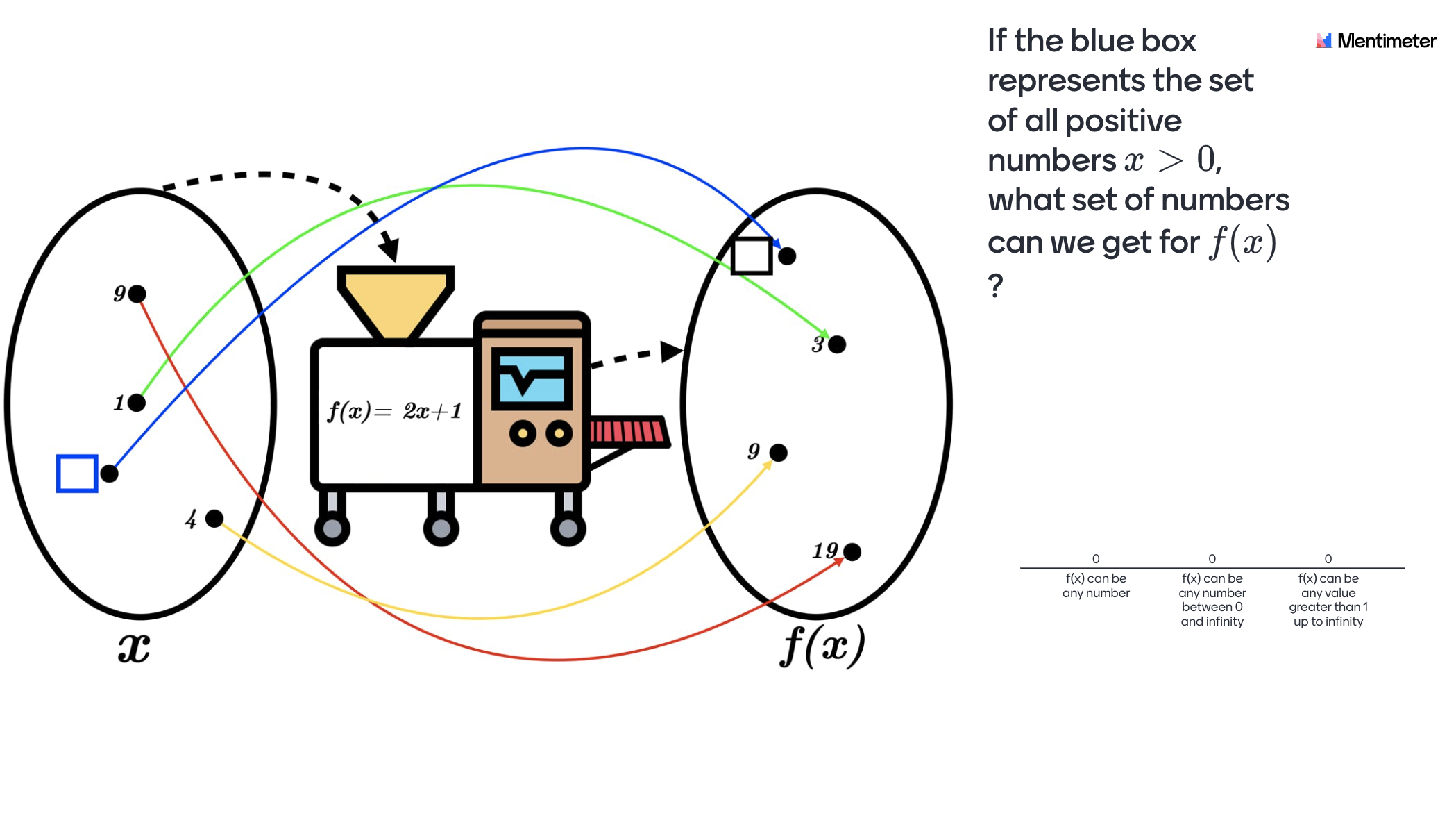Slide 12 - Formal definition: Give the students a definition that they (hopefully) can grasp and make sense to them at this point, and give two examples to make it less abstract.

Slide 13 - Three numbers machine: Give the students knowledge about how three different "inputs" correspond to precisely three unique outputs. In other words, a bijective function or '1-to-1' relation.

Slide 14 - The concept of a function: This is a key slide. The idea here is to give the students a visual representation of a linear function, where every input corresponds to a unique output. Try asking probing questions to the students, even if every group has answered correctly.

Slide 15 - Using the function with x as input: The purpose here is that the students should be accustomed to the mathematical notation of a function and be able to apply it.

Slide 16 - Going backwards: The purpose for the students here is to understand that functions and equations solving are interlinked.Slide 17 - Rank the different definitions of a function: Let the students individually rank the alternatives in order for them to decide how they conceive a function.

Slide 18 - Criteria for success revisited: Return to the problem that was introduced in the beginning of the lecture to make the students realize they’ve actually learnt something.

Slide 19 - Q&A: Let the students individually write down what they still feel is unclear about the concept of a function.

Slide 20-26 - Quiz Competition: Ask the students to enter their real names. Let the students compete in a fun way while you as a teacher can get a sense of the individual level of knowledge. This information could be used to give support for students in need of guidance.